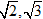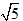## Class 10-Maths

#### Class 10-Maths

Course Language : English

Total Duration : 81 Hours

Course Price : ₹ 7000

Mathematics is considered as an Independent branch which is used by all branches of Science

NCERT Class 10 Maths Syllabus

UNIT I: NUMBER SYSTEMS

1. Real Numbers  (15 Periods)

Euclid’s division lemma, Fundamental Theorem of Arithmetic - statements after  reviewing work done earlier and after illustrating and motivating through examples, Proofs of irrationality ofDecimal representation of rational numbers in terms of terminating/non-terminating recurring decimals.

UNIT II: ALGEBRA

1. Polynomials  (7 Periods)

Zeros of a polynomial. Relationship between zeros and coefficients of quadratic polynomials. Statement and simple problems on division algorithm for polynomials with real coefficients.

2. Pair of Linear Equations in Two Variables (15 Periods)

Pair of linear equations in two variables and graphical method of their solution, consistency/inconsistency.

Algebraic conditions for number of solutions. Solution of a pair of linear equations in two variables algebraically - by substitution, by elimination and by cross multiplication method. Simple situational problems. Simple problems on equations reducible to linear equations.

Standard form of a quadratic equation ax2 + bx + c = 0, (a ≠ 0). Solutions of quadratic equations (only real roots) by factorization, and by using quadratic formula. Relationship between discriminant and nature of roots.

Situational problems based on quadratic equations related to day to day activities to be incorporated.

4. Arithmetic Progressions (8 Periods)

Motivation for studying Arithmetic Progression Derivation of the nth term and sum of the first n terms of an A.P. and their application in solving daily life problems.

UNIT III: COORDINATE GEOMETRY

1. Lines (In two-dimensions) (14 Periods)

Review: Concepts of coordinate geometry, graphs of linear equations. Distance formula. Section formula (internal division). Area of a triangle.

UNIT IV: GEOMETRY

1. Triangles (15 Periods)

Definitions, examples, counter examples of similar triangles.

1. (Prove) If a line is drawn parallel to one side of a triangle to intersect the other two sides in distinct points, the other two sides are divided in the same ratio.

2. (Motivate) If a line divides two sides of a triangle in the same ratio, the line is parallel to the third side.

3. (Motivate) If in two triangles, the corresponding angles are equal, their corresponding sides are proportional and the triangles are similar.

4. (Motivate) If the corresponding sides of two triangles are proportional, their corresponding angles are equal and the two triangles are similar.

5. (Motivate) If one angle of a triangle is equal to one angle of another triangle and the sides including these angles are proportional, the two triangles are similar.

6. (Motivate) If a perpendicular is drawn from the vertex of the right angle of a right triangle to the hypotenuse, the triangles on each side of the perpendicular are similar to the whole triangle and to each other.

7. (Prove) The ratio of the areas of two similar triangles is equal to the ratio of the squares of their corresponding sides.

8. (Prove) In a right triangle, the square on the hypotenuse is equal to the sum of the squares on the other two sides.

9. (Prove) In a triangle, if the square on one side is equal to sum of the squares on the other two sides, the angles opposite to the first side is a right angle.

2. Circles (8 Periods)

Tangent to a circle at, point of contact.

1. (Prove) The tangent at any point of a circle is perpendicular to the radius through the point of contact.

2. (Prove) The lengths of tangents drawn from an external point to a circle are equal.

3. Constructions (8 Periods)

1. Division of a line segment in a given ratio (internally).

2. Tangents to a circle from a point outside it.

3. Construction of a triangle similar to a given triangle.

UNIT V: TRIGONOMETRY

1. Introduction to Trigonometry (10 Periods)

Trigonometric ratios of an acute angle of a right-angled triangle. Proof of their existence (well defined); motivate the ratios whichever are defined at 0o and 90o. Values (with proofs) of the trigonometric ratios of 30o, 45o and 60o. Relationships between the ratios.

2. Trigonometric Identities (15 Periods)

Proof and applications of the identity sin2A + cos2A = 1. Only simple identities to be given. Trigonometric ratios of complementary angles.

3.  Heights and Distances: Angle of elevation, Angle of Depression. (8 Periods)

Simple problems on heights and distances. Problems should not involve more than two right triangles. Angles of elevation / depression should be only 30o, 45o, 60o.

UNIT VI: MENSURATION

1. Areas Related to Circles (12 Periods)

Motivate the area of a circle; area of sectors and segments of a circle. Problems based on areas and perimeter / circumference of the above said plane figures. (In calculating area of segment of a circle, problems should be restricted to central angle of 60°, 90° and 120° only. Plane figures involving triangles, simple quadrilaterals and circle should be taken.)

2. Surface Areas and Volumes (12 Periods)

1. Surface areas and volumes of combinations of any two of the following: cubes, cuboids, spheres, hemispheres and right circular cylinders/cones. Frustum of a cone.

2. Problems involving converting one type of metallic solid into another and other mixed problems. (Problems with combination of not more than two different solids be taken).

UNIT VII: STATISTICS AND PROBABILITY

1. Statistics (18 Periods)

Mean, median and mode of grouped data (bimodal situation to be avoided). Cumulative frequency graph.

2. Probability (10 Periods)

Classical definition of probability. Simple problems on single events (not using set notation).

#### Jobs

Mathematics is considered as an Independent branch which is used by all branches of Science

Algebra is one of the core subjects of mathematics. Algebra consists of the study of variables within number systems, along with operations that act on numbers and symbols. Wolfram|Alpha is a tremendous resource for solving equations; exploring polynomials; and studying fields, groups, vectors and matrices.

Arithmetic, branch of mathematics in which numbers, relations among numbers, and observations on numbers are studied and used to solve problems

Arithmetic (a term derived from the Greek word arithmos, “number”) refers generally to the elementary aspects of the theory of numbers arts of mensuration (measurement), and numerical computation (that is, the processes of addition, subtraction, multiplication, division, raising to powers, and extraction of roots).

Geometry is a Ancient Greek word. Geo- means "earth", -metron means "measurement") is a branch of mathematics concerned with questions of shape, size, relative position of figures, and the properties of space.

Gemoetry is study of objects in different shapes that include triangle, square, rectangle & other entities prevalent in the universe.

Triangles

The plane figure bounded by the union of three lines, which join three non collinear points, is called a triangle.
There are several special types of triangles with important properties.  But one property that all triangles share is that the sum of the lengths of any two of the sides is greater than the length of the third side or difference of the lengths of any two of the sides is less than the length of the third side, as illustrated below.

Triangles are similar if they have the same shape, they may be of different sizes. Therefore, the triangle obtained by rotating a triangle is similar to the original triangle. Similarly, the mirror image or the water image of a particular triangle is similar to the original triangle.
A triangle is defined by particularly six measures (three angles and three sides). But we don't need to know all of these to show that two triangles are similar to each other.

Triangles are similar if:

1. AAA (angle angle angle): Two triangles are said to be similar if two pairs of corresponding angles are equal. This also means that the remaining pair of the angles will be congruent as well.
2. SSS (side side side): Two triangles are also said to be similar if, all the three pairs of corresponding sides are in the same proportion to each other.
3. SAS (side angle side): Two triangles are said to be similar if, two pairs of sides are in the same proportion and the included angles are equal to each other.

When two triangles are similar, the following properties always hold true:

• Corresponding angles are equal to each other (same measure)
• Corresponding sides are in the same proportion to each other

Circles :

A circle is a set of points in a plane that are all located at the same distance from a fixed point (the center of the circle).
A chord of a circle is a line segment that has its endpoints on the circle.  A chord that passes through the center of the circle is a diameter of the circle.  A radius of a circle is a segment from the center of the circle to a point on the circle.  The words "diameter" and "radius" are also used to refer to the lengths of these segments.

Polygons :

A polygon is a closed figure formed by three or more than three line segments. These line segments are called the sides of the polygon. Each side of the polygon intersects exactly two other sides at their respective endpoints. The points of intersection of the sides of the polygon are known as vertices of the polygon. The term "polygon" is used to refer to a convex polygon, that is, a polygon in which each interior angle has a measure of less than 180.

Convex polygon: A type of polygon in which none of the interior angles is more than 180, is called a convex polygon.

Concave polygon: A type of polygon in which at least one of the interior angles of the polygon is more than 180, is called concave polygon.

Regular polygon: A type of polygon which has all its angles and sides equal is called a regular polygon.

A polygon with three sides is called a triangle; with four sides, is called a quadrilateral; with five sides, is called a pentagon; and with six sides, is called a hexagon.
The sum of the all the interior angles of a triangle is always 180.
The sum of all the interior angles of any polygon with n sides is equal to (n - 2)180.  For example, the sum for a hexagon is (6 - 2)180 = (4)180 = 720.

Note that, a hexagon can be divided into four triangles, therefore, the sum of the angle measures of a polygon can be found by adding the sum of the angle measures of four triangles. The perimeter of any polygon is the sum of the lengths of its sides. The phrase "area of a triangle" (or area of any other plane figure) is used to mean the area of the region enclosed by that particular figure.

Properties of polygons

• If number of sides of the polygon is n, then the sum of all the interior angles = (n-2)180.
• Interior angle of a polygon + corresponding exterior angle of that polygon = 180.
• The sum of all the exterior angles of a polygon = 360°.
• The number of the  diagonals in a polygon of n sides is always  = (n(n-1)/2) - 1
###### Student Reviews